#2019 iT 邦幫忙鐵人賽DAY 27
1

## [2019鐵人賽Day27]老蕭咖啡館-逐漸揭開的真相(特調的感觸篇)（Calculator計算機基本運算元件功能製作）

（林宇拿起霏語的杯子輕啜了一口）

（林宇再度嚐了一口，並閉上了眼去回想剛剛的種種）

（她們說話的同時，我也再調製了一杯給霏語）

（我倆對望微笑著看著對方）

（過了一會，林宇睜開眼，但卻看到她眼角流下淚水）

（林宇像個孩子一樣的在霏語懷裏哭泣）

（霏語狠狠地白了我一眼）

（林宇）

（突然，霏語轉向我微笑，這一刻好美，非常的美，美得讓人想把這一刻停下，不再向前）

（過了一會）

（林宇）

（突然被兩人瞪了一下）

（本故事純屬虛構，如有雷同實屬巧合）

「簡易計算機(基本運算元件功能製作)」
Step1. 製作數字按鈕功能(當畫面為0或運算符號時，後方不會直接加上數字)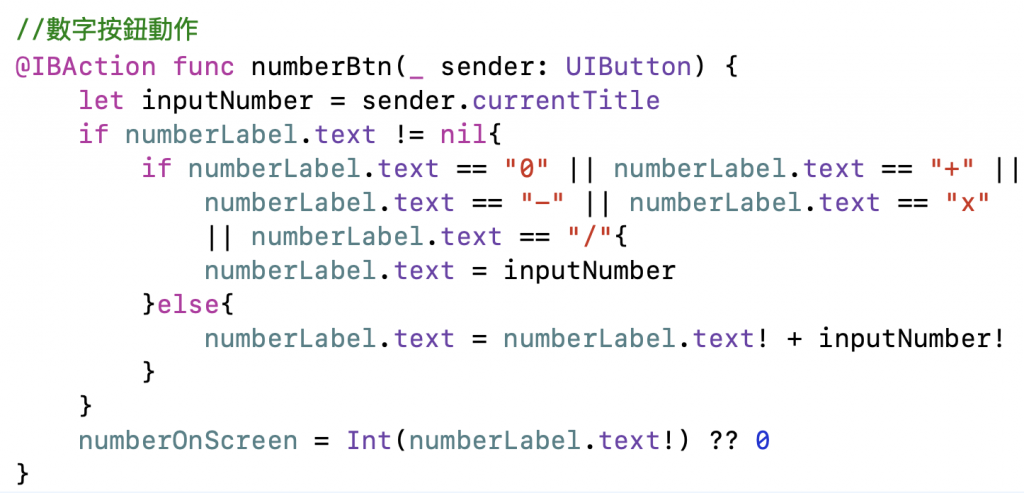``````//數字按鈕動作
@IBAction func numberBtn(_ sender: UIButton) {
let inputNumber = sender.currentTitle
if numberLabel.text != nil{
if numberLabel.text == "0" || numberLabel.text == "+" || numberLabel.text == "-" || numberLabel.text == "x" || numberLabel.text == "/"{
numberLabel.text = inputNumber
}else{
numberLabel.text = numberLabel.text! + inputNumber!
}
}
numberOnScreen = Int(numberLabel.text!) ?? 0
}
``````

Step2. 增加運算模式列舉，將於等於時判斷是何種模式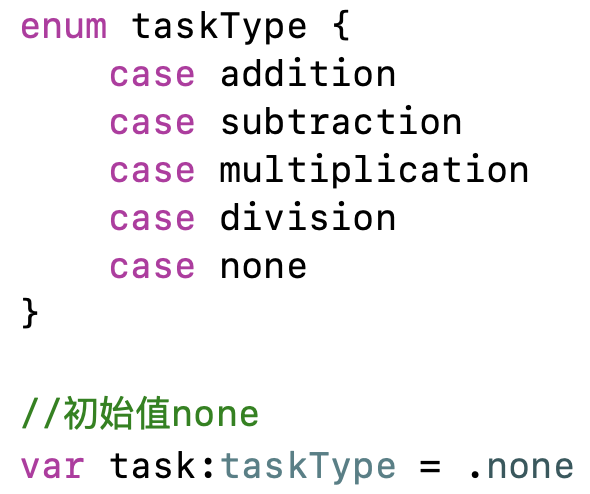``````enum taskType {
case subtraction
case multiplication
case division
case none
}

//初始值none
``````

Step3. 製作AC清空按鈕功能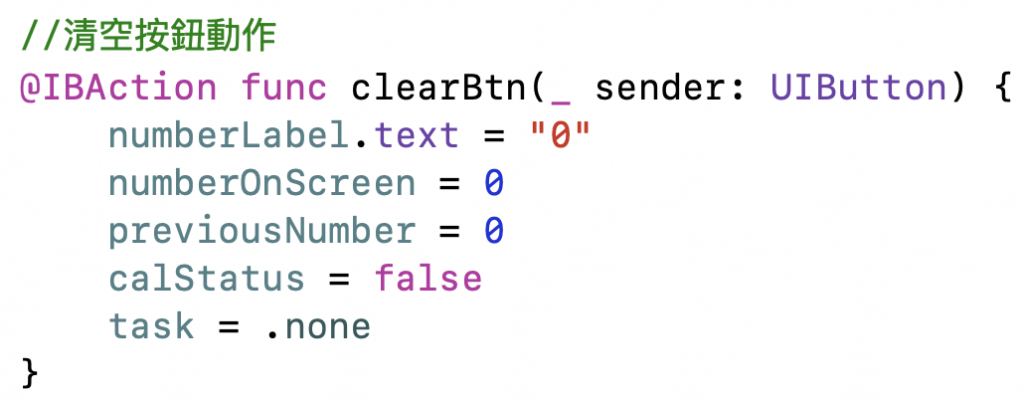``````//清空按鈕動作
@IBAction func clearBtn(_ sender: UIButton) {
numberLabel.text = "0"
numberOnScreen = 0
previousNumber = 0
calStatus = false
}
``````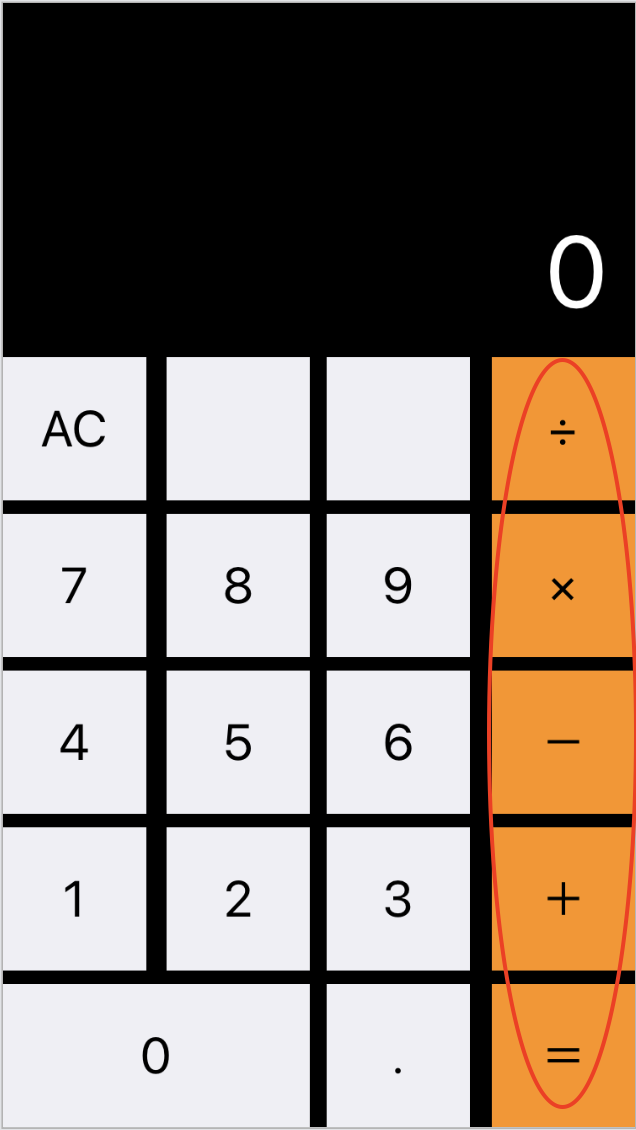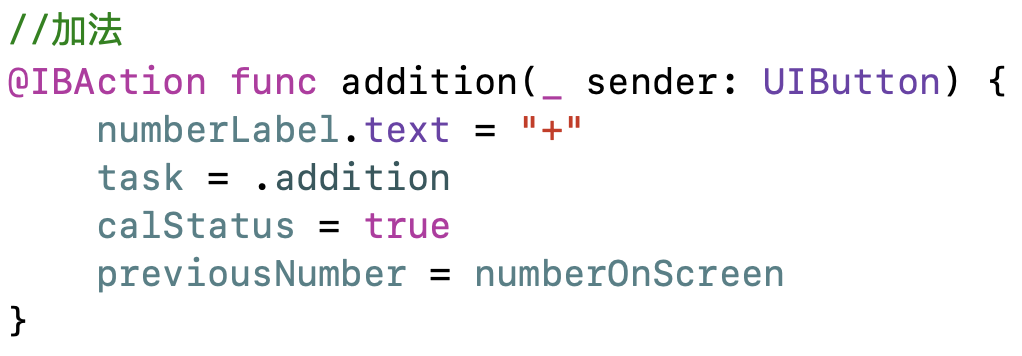``````//加法
@IBAction func addition(_ sender: UIButton) {
numberLabel.text = "+"
calStatus = true
previousNumber = numberOnScreen
}
``````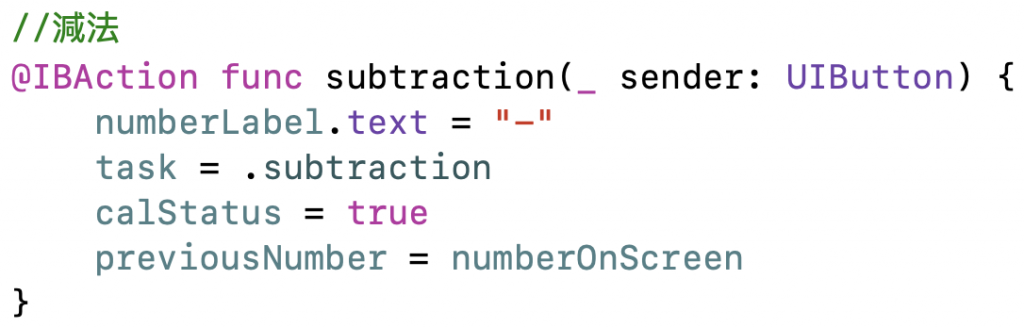``````//減法
@IBAction func subtraction(_ sender: UIButton) {
numberLabel.text = "-"
calStatus = true
previousNumber = numberOnScreen
}
``````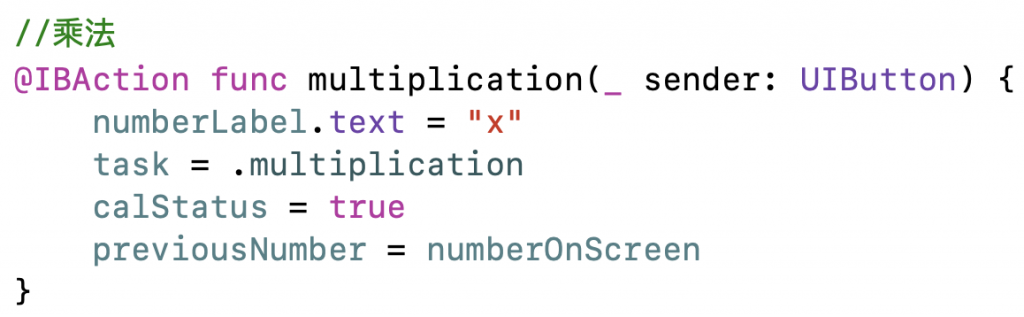``````//乘法
@IBAction func multiplication(_ sender: UIButton) {
numberLabel.text = "x"
calStatus = true
previousNumber = numberOnScreen
}
````````````//除法
@IBAction func division(_ sender: UIButton) {
numberLabel.text = "/"